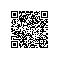# 第13周报告1：用函数完成分段数学函数的求解

0                           当x<0.3时;

f(x)=  (x-0.3)/(0.8-0.3)  当0.3≤x≤0.8时

1                             当x>0.8时

/*
* 程序的版权和版本声明部分
* All rightsreserved.
* 文件名称： fun.cpp
* 作    者： 贺利坚
* 完成日期： 2011 年 11 月 22  日
* 版本号： v1.0

* 对任务及求解方法的描述部分
* 输入描述：函数自变量x
* 问题描述：求函数的值：当x<0.3时，f(x)=0; 当0.3≤x≤0.8时，f(x)=(x-0.3)/(0.8-0.3);当x>0.8时，f(x)=1;
* 程序输出：f(x)的值
*/
#include<iostream>
using namespacestd;
const doublex1=0.3, x2=0.8; //定义为全局常变量，方便，易维护
doublef(double);     //此处加入对自定义函数f的声明

int main( )
{
double x;
cout<<"请输入x的值：";
cin>>x;
cout<<"解得：f("<<x<<")="<<f(x)<<"\n";
return 0;
}
//下面是自定义函数的定义部分
//当x<0.3时，f(x)=0; 当0.3≤x≤0.8时，f(x)=(x-0.3)/(0.8-0.3);当x>0.8时，f(x)=1;

double f(doublex)
{
double result;
if(x<x1)
result=0;
else if (x>x2)
result=1;
else
result = (x-x1)/(x2-x1);
return result;
}

(1) 遵循“判定/条件测试”覆盖原则（设计足够的测试用例，使得判定表达式的每个条件的所有可能取值至少出现一次，并使每个判定表达式所有可能的结果也至少出现一次），我设计了三组测试用例，测试方案及结果如下

1 x=0.2 0 0 正确
2 x=0.5 0.4 0.4 正确
3 x=0.9 1 1 正确

(2)判定的边界值是测试的“敏感”区，针对本程序的两个边界值，我设计了下面的两组测试用例，测试方案及结果如下

4 x=0.3 0 0 正确
5 x=0.8 1 1 正确

经验积累：

1. 程序的测试也是技术活，需要考虑各种可能的情况，设计用于测试的输入，将执行结果与预期的结果对比后才能得出结论。程序工作并不是能输出结果（不管对错）就视为完成。

2. 布置题目时，专门选择了以前做过的题目，同学们只要专心写函数而不用多想算法。用心良苦，感动ing

3. 同学们做的不错，几乎全做出来了，并且真的将函数中的几个概念通过这个题搞清楚了。使用钉钉扫一扫加入圈子
+ 订阅